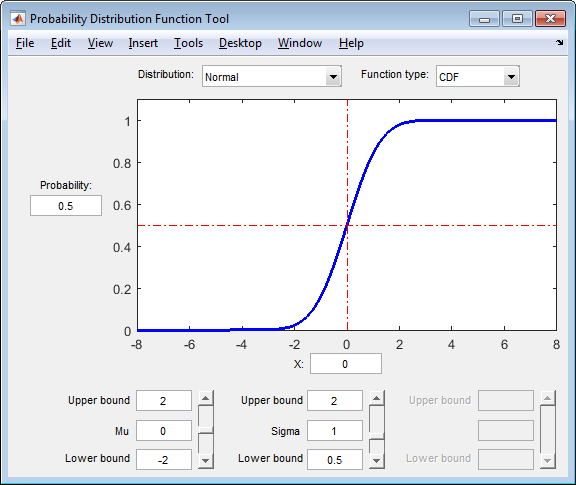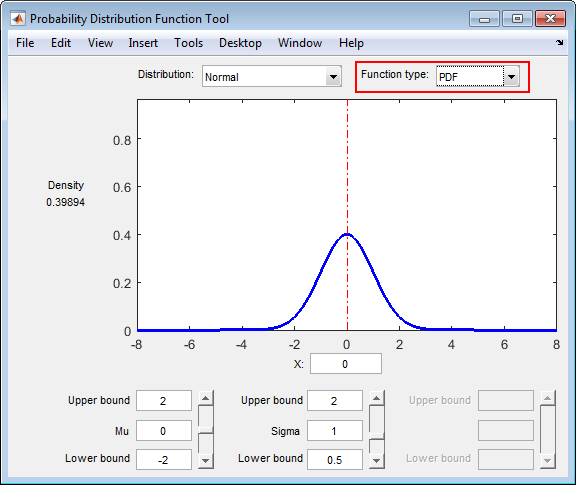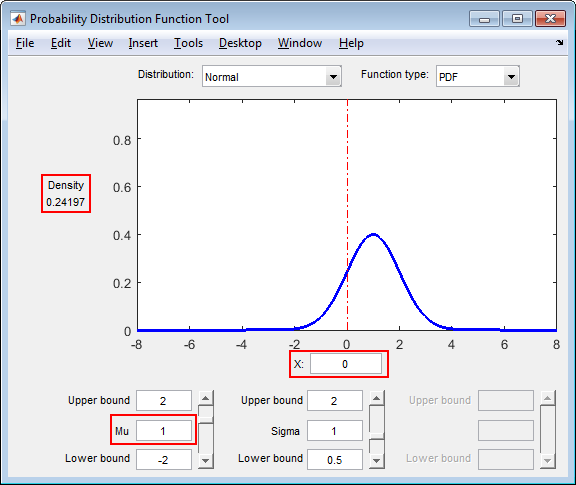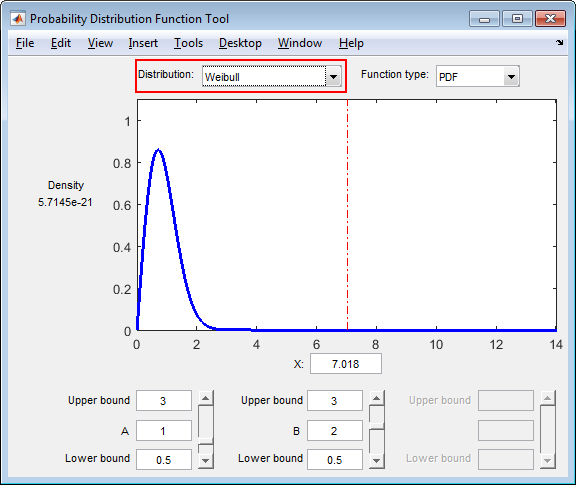# Probability Distribution Function

Interactive density and distribution plots

## Description

The Probability Distribution Function user interface creates an interactive plot of the cumulative distribution function (cdf) or probability density function (pdf) for a probability distribution. Explore the effects of changing parameter values on the shape of the plot, either by specifying parameter values or using interactive sliders.

### Required Products

• MATLAB®

• Statistics and Machine Learning Toolbox™

Note: `disttool` does not provide printing, code generating, or data importing functionality in MATLAB Online™.

## Open the Probability Distribution Function App

• At the command prompt, enter `disttool`.

## Examples

expand all

This example shows how to use the Probability Distribution Function user interface to explore the shape of cdf and pdf plots for different probability distributions and parameter values.

Open the Probability Distribution Function user interface.

`disttool`The interface opens with a plot of the cdf of the Normal distribution. The initial parameter settings are `Mu = 0` and ```Sigma = 1```.

Select `PDF` from the Function type drop-down menu to plot the pdf of the Normal distribution using the same parameter values.Change the value of the location parameter `Mu` to 1.As the parameter values change, the shape of the plot also changes. Also, the value of `X` remains the same, but the density value changes because of the new parameter value.

Use the Distribution drop-down menu to change the distribution type from `Normal` to Weibull.The shape of the plot changes, along with the names and values of the parameters.

## Parameters

Specify the probability distribution to explore by selecting a distribution name from the drop-down list. The drop-down list includes approximately 25 probability distribution options, including `Normal`, `Exponential`, `Poisson`, `Weibull`, and more.

Specify the probability distribution function type as `CDF` (cumulative distribution function) or `PDF` (probability density function) by selecting the function name from the drop-down list.

Specify the cumulative distribution function (cdf) value of interest as a numeric value in the range [0,1]. The corresponding random variable value appears in the X field below the plot. Alternatively, you can specify a value for X, and the Probability value will update automatically.

This option only appears if Function type is `CDF`. If Function type is `PDF`, then the probability density at the specified X value displays to the left of the plot.

Specify the random variable of interest as a numeric value. If the Function type is `CDF`, then the corresponding cumulative distribution function (cdf) value appears in the Probability field to the left of the plot. Alternatively, you can specify a value for Probability, and the X value will update automatically. If the Function type is `PDF`, then the corresponding probability density value appears to the left of the plot.

Specify the parameter boundaries and values as numeric values. Each column contains a field for the upper bound, value, and lower bound of one parameter. The name and number of available parameters changes based on the distribution specified in the Distribution drop-down list. For example, if you select the `Normal` distribution, then `disttool` enables two columns: One column for the Mu parameter and one column for the Sigma parameter. If you select the Exponential distribution, then `disttool` enables one column for the Mu parameter.

## Tips

To change the value of X (on the y-axis), or Probability or Density (on the x-axis):

• Type the values of interest into the Probability or X fields;

• Click on the point of interest on the plot; or

• Click and drag the reference lines across the plot.